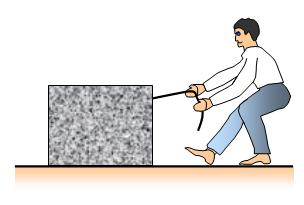# WorkA rocket accelerating upwards
A heavy box being pushed across the floor
Someone lift up a weight
A car pulls a caravan
A car braking to a stop
A ball being kicked
A train moving along the track

These are all examples of WORK and ENERGY

When we lift up a weight, stretch a piece of elastic or push a box across the floor we are doing WORK and changing energy from one form to another. In each case we are pushing or pulling against a force, in the first case gravity and in the second case the tension of the elastic and so on. The further we lift or pull and the bigger the force we use the more work we do. When a car brakes to a stop work is being done by the frictional forces in the brakes and between the wheels and the road. If a clockwork motor is used to lift up a stone then the energy stored in the wound up spring is changed into stored energy of the stone. (We called this gravitational potential energy). The stone has been raised up against the force of gravity and so work has been done on it.

When work is done a force is moved through a certain distance and the formula for the work done is:

Work done (W) = Force used (F) x distance moved (d)

The units for work are JOULES with the force in NEWTONS and the distance in METRES.

1 JOULE of work is done when a force of 1 NEWTON is moved a distance of 1 METRE.

Example problems
1. A heavy box is pulled across a horizontal floor by a force of 20 N. If it is moved 4 m how much work has been done?
Work done = force x distance = 20x4 = 80J

2. A mass of 3 kg is lifted vertically through a distance of 5m. How much work has been done?
Gravitational intensity = 10 N/kg.
Force on the 3kg mass = its weight = 10x3 = 30N
Work done = force x distance = 30x5 = 150 J

When work is done energy must be changed from one form to another. This work is a direct result of the energy change and so energy is measured in the same units as work – joules. If 48J of work are done then 48J of energy are changed from one form of energy to others.

Note: Strictly speaking the formula should also mention the direction of the force. Work is force x distance moved in the direction of the force. Therefore for a planet going round the Sun no work is being done because the gravitational force of the Sun is always at right angles to the motion of the planet.

For further details and a set of work sheets see: Forces and energy

A VERSION IN WORD IS AVAILABLE ON THE SCHOOLPHYSICS CD

© Keith Gibbs 2013